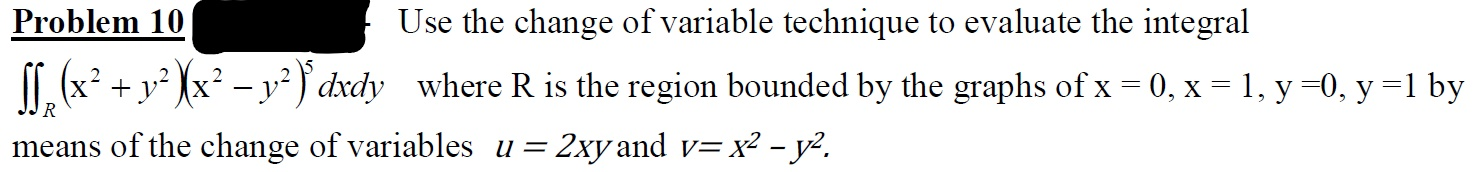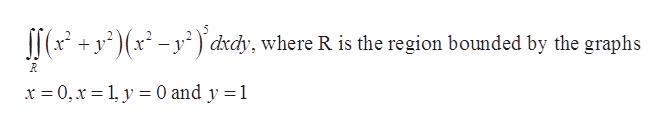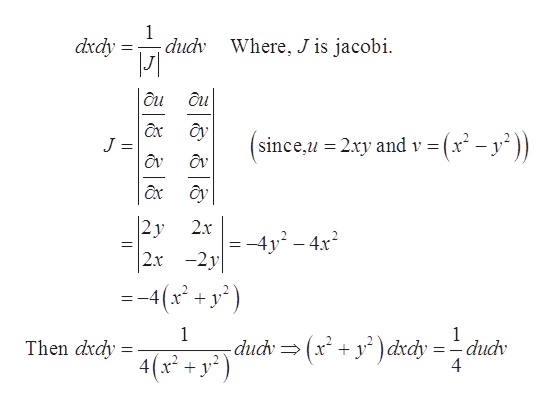# Problem 10Use the change of variable technique to evaluate the integralT. (x² + y² Xx² – y² } dxdywhere R is the region bounded by the graphs of x = 0, x = 1, y =0, y =1 byR= 2xy and v= x² - y².means of the change of variables u =

Question
4 viewshelp_outlineImage TranscriptioncloseProblem 10 Use the change of variable technique to evaluate the integral T. (x² + y² Xx² – y² } dxdy where R is the region bounded by the graphs of x = 0, x = 1, y =0, y =1 by R = 2xy and v= x² - y². means of the change of variables u = fullscreen
check_circle

star
star
star
star
star
1 Rating
Step 1

Given integral and region R are,help_outlineImage Transcriptionclose|(* +y° )(x² - y²) dxdy, where R is the region bounded by the graphs x = 0, x = 1, y = 0 and y = 1 fullscreen
Step 2

Given the values of u and v as,

Step 3

Now, changing the v...help_outlineImage Transcriptionclosedudv Where, J is jacobi. dxdy ôu ôu ây J = since,u = 2xy and v = ôx ây 2y 2x -2y 2.x -4y - 4x = -4(x + y²) dudv= (x + y* ) dxdy = - dudv 4 Then dxdy = 4(x* + y²) || fullscreen

### Want to see the full answer?

See Solution

#### Want to see this answer and more?

Solutions are written by subject experts who are available 24/7. Questions are typically answered within 1 hour.*

See Solution
*Response times may vary by subject and question.
Tagged in

### Math